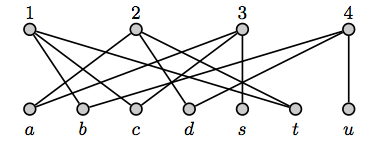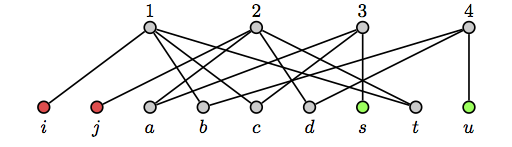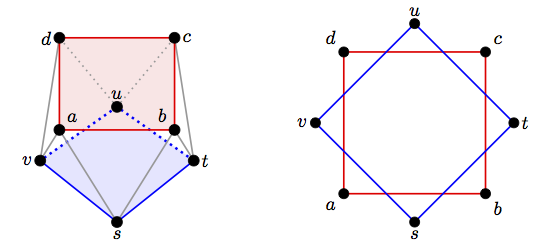# $P_{8}^{=}$ is not a gammoid

Guest post by Joe Bonin.

In his talk at the recent workshop in Eindhoven, Immanuel Albrecht noted that each matroid in the appendix of examples in James Oxley’s book Matroid Theory is designated as either being a gammoid or not, except for $P_8^=$. In this post, we show that $P_8^=$ is not a gammoid. The ideas used may apply more widely.

# Transversal and cotransversal matroids

Gammoids are minors of transversal matroids, so in this section, we sketch the items about transversal matroids and their duals that we use. Those who know the characterization of transversal and cotransversal matroids in Theorems 2 and 3 might prefer to omit this introductory section.

We start with a bipartite graph with vertex classes $S$ and $T$. We will use the example below, with $S=\{a,b,c,d,s,t,u\}$.A partial transversal is a subset of $S$, such as $\{a,b,u\}$ above, that can be matched with a subset of the vertices in $T$ via edges in the graph. The partial transversals are the independent sets of the resulting transversal matroid.

For $X\subseteq S$, let $N_G(X)$ denote the set of neighbors of $X$ in $G$, that is,
$$N_G(X) = \{v\in T\,:\, v \text{ is adjacent to at least one } x\in X\}.$$ A set $X$ in a matroid $M$ is cyclic if $X$ is a union of circuits, that is, $M|X$ has no coloops. The cyclic flats in the example are $\emptyset$, $\{a,c,s,t\}$, $\{b,d,t,u\}$, $\{a,d,s,u\}$, $\{b,c,s,u\}$, and $S$. In this example, the rank of each cyclic flat ($0$, $3$, $3$, $3$, $3$, and $4$, respectively) is its number of neighbors. This illustrates the lemma below.

Lemma 1. Let $G$ be a bipartite graph on $S\cup T$ that represents $M$. If $X$ is a cyclic set of rank $k$ in $M$, then $|N_G(X)|=k$.

Proof. A basis of $X$ can be matched to the vertices in some $k$-element subset $T’$ of $T$. Let $G’$ be the induced subgraph of $G$ on $S\cup T’$, so $G’$ gives a rank-$k$ transversal matroid $M’$ on $S$, and $r_{M’}(X)=k$.

We first show that $M’|X=M|X$. Independent sets in $M’|X$ are independent in $M|X$. If the converse failed, then some circuit $C$ of $M’|X$ would be independent in $M|X$. Since $C$ can be matched in $G$ but not in $G’$, some $a\in C$ is adjacent to a vertex $v\in T-T’$. Extend $C-a$ to a basis $B$ of $M’|X$. Now $r_{M’}(X)=k$, so matching $B$ with the vertices in $T’$ and $a$ with $v$ gives the contradiction $r_M(X)>k$. Thus, $M’|X=M|X$.

We now get that $N_G(X)=T’$, for if instead some $b\in X$ were adjacent to some vertex $v\in T-T’$, then the same type of matching argument, using a basis $B$ of $M|X$ with $b\not\in B$ (which exists since $b$ is not a coloop of $M|X=M’|X$), would yield a contradiction. Thus, $|N_G(X)|=k$. $\square$

This proof adapts to show that we can always choose $G$ so that $|T|=r(M)$.

The bipartite graph $G$ on $S\cup T$ is an induced subgraph of a bipartite graph $G’$ on $S’\cup T$ in which, for each $x\in T$, there is a $y\in S’$ with $N_{G’}(y)=\{x\}$. Such an extension of our example above is shown below; the red vertices have been added.

The bipartite graph $G$ on $S\cup T$ is an induced subgraph of a bipartite graph $G’$ on $S’\cup T$ in which, for each $x\in T$, there is a $y\in S’$ with $N_{G’}(y)=\{x\}$. Such an extension of our example above is shown below; the red vertices have been added.The resulting matroid $M’$ is an extension of $M$.

Pick a subset $B$ of $S’$ (for example, the red and green vertices above) for which, for each $x\in T$, there is one $y\in B$ with $N_{G’}(y)=\{x\}$. Clearly $B$ is a basis of $M’$. Moreover, if $X$ is a cyclic flat of $M’$, then $X\cap B$ is a basis of $X$ since $r(X) = |N_{G’}(X)|$ by Lemma 1, so the flat $X$ must contain the elements of $B$ that are adjacent to the vertices in $N_{G’}(X)$. Thus, $r_{M’}(X) = |X\cap B|$. It is not hard to show, more generally, that for any cyclic flats $X_1,X_2,\ldots,X_n$ of $M’$,
\begin{equation*}
r_{M’}(X_1\cup X_2\cup \cdots \cup X_n )= \bigl|B\cap (X_1\cup X_2\cup
\cdots \cup X_n)\bigl|
\end{equation*}
and
\begin{equation*}
r_{M’}(X_1\cap X_2\cap \cdots \cap X_n) = \bigl|B\cap (X_1\cap X_2\cap
\cdots \cap X_n)\bigr|.
\end{equation*}
From these equations and inclusion/exclusion, it follows that
\begin{equation*}
r_{M’}(X_1\cap X_2\cap \cdots \cap X_n) =
\sum_{J\subseteq[n]} (-1)^{|J|+1}r_{M’}\bigl(\bigcup_{j\in J}X_j\bigr).
\end{equation*}

A transversal matroid might not have the type of basis that we used to derive the equalities above, but we do get the inequality in Theorem 2 below. To see this, delete $S’-S$ to get the original transversal matroid $M$. The rank of unions of cyclic flats of $M$ are the same as for their closures in $M’$, but the rank of intersections may be less. This proves the half of Theorem 2 that we will use. (For a proof of the converse, see .) John Mason proved this result for cyclic sets and Aubrey Ingleton refined it to cyclic flats. We let $\cup\mathcal{F}$ denote the union of a family of sets, and we use $\cap\mathcal{F}$ similarly.

Theorem 2. A matroid $M$ is transversal if and only if for all nonempty sets $\mathcal{A}$ of cyclic flats of $M$,
\begin{equation*}
r(\cap\mathcal{A})\leq \sum_{\mathcal{F}\subseteq \mathcal{A}} (-1)^{|\mathcal{F}|+1} r(\cup\mathcal{F}).
\end{equation*}

We will use the dual of this result, which we state next. Duals of transversal matroids are called cotransversal matroids or strict gammoids.

Theorem 3. A matroid $M$ is cotransversal if and only if for all sets $\mathcal{A}$ of cyclic flats of $M$,
\begin{equation}
r(\cup \mathcal{A}) \leq \sum_{\mathcal{F}\subseteq \mathcal{A}\,:\,\mathcal{F}\ne\emptyset}
\end{equation}

# Gammoids and $P_8^=$

Restrictions of transversal matroids are transversal, so any gammoid (a minor of a transversal matroid) is a contraction of a transversal matroid. The set we contract can be taken to be independent since $M/X =M\backslash (X-B)/B$ if $B$ is a basis of $M|X$, so any gammoid is a nullity-preserving contraction of a transversal matroid. The class of gammoids is closed under duality, so we get Lemma 4 below.

Lemma 4. Any gammoid has a rank-preserving extension to a cotransversal matroid.

Because of this lemma, below we focus exclusively on extensions that are rank-preserving.

We will also use the corollary below of the following theorem of Ingleton and Piff .

Theorem 5. A matroid of rank at most three is a gammoid if and only if it is cotransversal.

Corollary 6. Let $M$ be a rank-$r$ matroid with $r\geq 3$. If $H_1$, $H_2$, $H_3$, and $H_4$ are cyclic hyperplanes, any two of which intersect in a flat of rank $r-2$, and each set of three or four intersects in a flat $X$ of rank $r-3$, then $M$ is not a gammoid.

Proof. In $M/X$, the sets $H_i-X$, for $1\leq i\leq 4$, are cyclic lines. (Contraction does not create coloops.) The rank of the union of these four cyclic lines is $3$, but the alternating sum in inequality (1) is $4\cdot 2 – 6\cdot 1 = 2$, so $M/X$ is not cotransversal by Theorem 3. Thus, since $r(M/X)=3$, it is not a gammoid by Theorem 5, so $M$ is not a gammoid. $\square$

To show that $P_8^=$ is not a gammoid, we focus on a particular failure of inequality (1) in $P_8^=$ and show that between $P_8^=$ and any extension $M’$ of $P_8^=$ in which the counterpart of that particular inequality holds, we have a single-element extension of $P_8^=$ to which Corollary 6 applies. Thus, any such $M’$ has a restriction that is not a gammoid, so $M’$ is not cotransversal, and so $P_8^=$ is not a gammoid by Lemma 4.

To define $P_8^=$, we first briefly discuss $P_8$, which we get by placing points at the vertices of a cube and twisting the top of the cube an eighth turn. Label the points in the top and bottom planes of $P_8$ as shown below (the second diagram shows the view from above). We get $P_8^=$ by from $P_8$ by relaxing the circuit-hyperplanes $\{a,b,c,d\}$ and $\{s,t,u,v\}$.From this, we see that the cyclic flats of $P_8^=$, besides the empty set and the whole set, are the following planes. In each, we put what we call its diagonal in bold.
$$\{\mathbf{a},\mathbf{c},u,v\} \quad\{\mathbf{a},\mathbf{c},s,t\} \quad \{\mathbf{b},\mathbf{d},s,v\}\quad \{\mathbf{b},\mathbf{d},t,u\}$$
$$\{a,b,\mathbf{t},\mathbf{v}\} \quad\{c,d,\mathbf{t},\mathbf{v}\}\quad \{a,d,\mathbf{s},\mathbf{u}\}\quad \{b,c,\mathbf{s},\mathbf{u}\}$$

Observe that each cyclic plane $X$ intersects five others in exactly two points (this includes all four cyclic planes that are not in the same row as $X$), and the remaining two cyclic planes in one point each (and those are different points). Thus, the number of sets of two cyclic planes that intersect in two points is $8\cdot 5/2 = 20$, and the number of sets of two cyclic planes that intersect in a single point is $8\cdot 2/2 = 8$. No triple of cyclic planes intersects in two points. Also, each point is in exactly four cyclic planes, so the number of sets of three planes that intersect in a singleton is $8\cdot 4=32$, and the number of sets of four points that intersect in a singleton is $8$.

Let $\mathcal{A}$ be the set of all eight cyclic planes. The term on the left side of inequality (1) is $4$. On the right side, the counting in the previous paragraph gives $$8\cdot 3 – 20\cdot 2 -8 + 32 -8 = 0.$$ Thus, inequality (1) fails.

Let $M’$ be a rank-preserving extension of $P_8^=$ in which the counterpart of this instance of inequality (1) holds. (If there is no such $M’$, then $P_8^=$ is not a gammoid, as we aim to show.) Think of constructing $M’$ by a sequence of single-element extensions. If each of these single-element extensions added a point to at most two of the cyclic planes of $P_8^=$, or parallel to a point of $P_8^=$, then the counterpart of this instance of inequality (1) would fail; thus, not all extensions are of these types. Focus on one point, say $e$, that is added to at least three cyclic planes of $P_8^=$ and not parallel to an element of $P_8^=$, and consider the single-element extension of $P_8^=$ formed by restricting $M’$ to $E(P_8^=)\cup e$. We show that, up to symmetry, there are only two such single-element extensions of $P_8^=$. As we see below, in both options, $e$ is added to exactly three cyclic planes, not more.

First consider adding $e$ to two cyclic planes that have the same diagonal, say, by symmetry, $\{a,c,u,v\}$ and $\{a,c,s,t\}$. We cannot add $e$ to $\{a,b,t,v\}$ since this plane intersects the other two in lines that share a point: adding $e$ to all three planes would give $r(\{a,v,e\})=2=r(\{a,t,e\})$, so either $e$ is parallel to $a$ (which we discarded) or $\{a,t,v\}$ would be a $3$-circuit, contrary to the structure of $P_8^=$. Similar reasoning eliminates adding $e$ to any plane in the second row. The only candidates left are $\{b,d,s,v\}$ and $\{b,d,t,u\}$, and we cannot add $e$ to both since then $\{a,c,e\}$ and $\{b,d,e\}$ would be lines that intersect in $e$, but $a,b,c,d$ are not coplanar.

Now assume that no two planes to which we add $e$ have the same diagonal. We must have a pair of sets in the same row but with different diagonals; by symmetry, we can use $\{a,c,u,v\}$ and $\{b,d,s,v\}$. An argument like the one above shows that the only other planes to which we can add $e$ are $\{a,d,s,u\}$ or $\{b,c,s,u\}$, and we cannot add $e$ to both since they have the same diagonal.

Thus, between $P_8^=$ and $M’$ we have a single-element extension $M$ of $P_8^=$ in which a point $e$ is added to exactly three of the original cyclic planes. By the argument above, up to symmetry, there are two cases to consider: the extended cyclic planes are either

1. $\{a,c,u,v,e\}$,  $\{a,c,s,t,e\}$,  and $\{b,d,s,v,e\}$,  or
2. $\{a,c,u,v,e\}$, $\{b,d,s,v,e\}$, and $\{a,d,s,u,e\}$.

In either case, the cyclic planes of $M$ that contain $v$, that is, $$\{a,c,u,v,e\}, \quad \{b,d,s,v,e\},\quad \{a,b,t,v\}, \quad\{c,d,t,v\},$$ satisfy the hypothesis of Lemma 6, so $M$ is not a gammoid. Thus, no coextension of $M$ is cotransversal, so $P_8^=$ is not a gammoid. Indeed, we have the result below.

Proposition 7. The matroid $P_8^=$ is an excluded minor for the class of gammoids.

To prove this, first check that $P_8^=$ is self-dual. With that and the symmetry of $P_8^=$, it suffices to check that $P_8^=\backslash v$ is a gammoid. One can check that it is the transversal matroid in our example in the introductory section.

# References

 J. Bonin, J.P.S. Kung, and A. de Mier, Characterizations of transversal and fundamental transversal matroids, Electron. J. Combin. 18 (2011) #P106.

 A.W. Ingleton and M.J. Piff, Gammoids and transversal matroids, J. Combinatorial Theory Ser. B 15 (1973) 51-68.

This site uses Akismet to reduce spam. Learn how your comment data is processed.